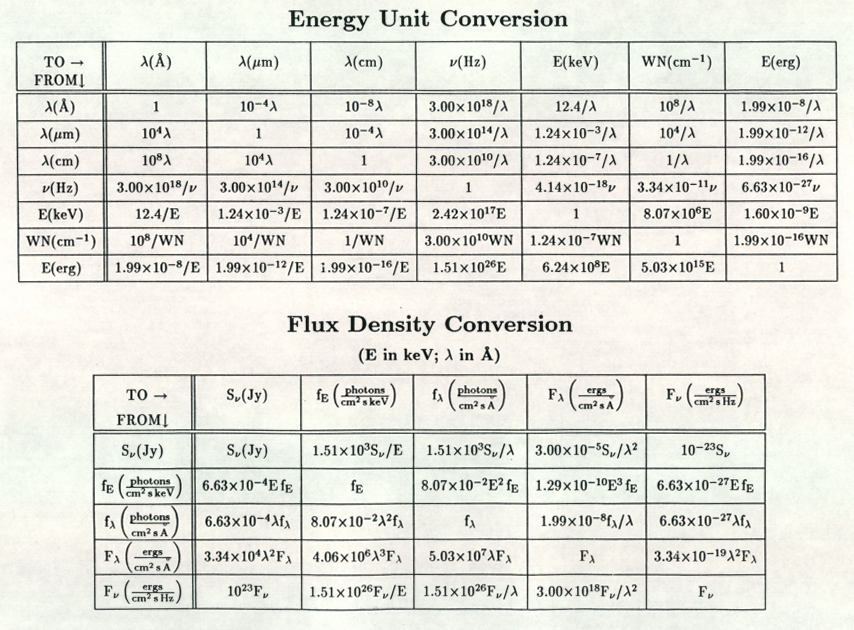# Fundamental astronomical conversions:

### Energy unit and Flux density conversions### Flux conversions:

[Y erg/cm^2/s] = 1000 * [X W/m^2]
[Y erg/cm^2/s/Hz] = 1000 * [X W/m^2/Hz]
[Y erg/cm^2/s/A] = 2.99792458E+21 * [X1 W/m^2/Hz] / [X2 A]^2
[Y W/m^2/um] = 2.99792458E+14 * [X1 W/m^2/Hz] / [X2 um]^2
[Y photon/cm^2/s/A] WRONG? = 1.50918896E+29 * [X1 W/m^2/Hz] / [X2 A]
[Y photon/cm^2/s/um] = 1.50918896E+22 * [X1 erg/cm^2/Hz] / [X2 um]
[Y photon/cm^2/s/um] = 5.03411250E+14 * [X1 W/m^2/um] * [X2 um]
[Y photon/cm^2/s/A] = 5.03411250E+07 * [X1 erg/cm^2/s/A] * [X2 A]
[Y Jy] = 1.0E+26 * [X W/m^2/Hz]
[Y Jy] = 1.0E+23 * [X erg/cm^2/s/Hz]
[Y Jy] = 3.33564095E+04 * [X1 erg/cm^2/s/A] * [X2 A]^2
[Y erg/cm^2/s/A] = 2.99792458E-05 * [X1 Jy] / [X2 A]^2
[Y photon/cm^2/s/A] = 1.50918896E+03 * [X1 Jy] / [X2 A]
[Y S10] = log([6.86E-06 * X erg/s/sr]) @ 5500 A
[Y S10] ?= log([3.77E-02 * X erg/s/Hz/sr] / [5500 A ])
[Y S10] = [X 10th mag A0 V stars / deg^2]
[Y S10] = 10^(-0.4([X B surface brightness] – 27.78))

### Surface brightness conversions:

[Y photon/cm^2/s/A/sr] = 7.9577539E+04 [X Rayleigh]
[Y photon/cm^2/s/A/deg^2] = 2.4240705E+01 [X Rayleigh]
[Y photon/cm^2/s/A/arcsec^2] = 1.8704247E-06 [X Rayleigh]
[Y photon/cm^2/s/A/arcsec^2] = 3.54726271E+18 * [X1 W/m^2/Hz/sr] / [X2 A]
[Y photon/cm^2/s/A/arcsec^2] = 5.03411250E+04 * [X1 erg/cm^2/s/Hz/sr] / [X2 A]
[Y U surface brightness] = -2.5 * log[X1 erg/cm^2/s/Hz/sr] – 22.78
[Y B surface brightness] = -2.5 * log[X1 erg/cm^2/s/Hz/sr] – 21.85
[Y V surface brightness] = -2.5 * log[X1 erg/cm^2/s/Hz/sr] – 22.02
[Y R surface brightness] = -2.5 * log[X1 erg/cm^2/s/Hz/sr] – 22.21
[Y I surface brightness] = -2.5 * log[X1 erg/cm^2/s/Hz/sr] – 22.41